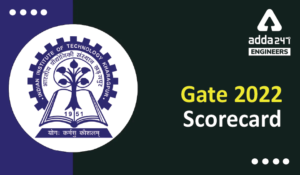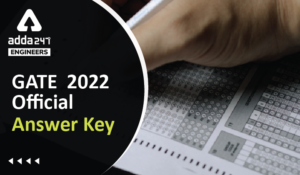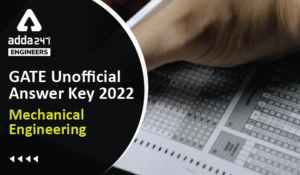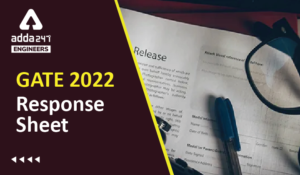Engineering Jobs   »   Electrical Engineering quizs   »   GATE 2022, ELECTRICAL GATE QUIZ,

# GATE’22 EE: Daily Practices Quiz 17-Sep-2021

## GATE 2022 Important Dates

Organizing Institute for GATE 2022 is the Indian Institute of Technology Kharagpur. For GATE 2022 Exam IIT Kharagpur has released the official notification and Important dates regarding the GATE 2022 Exam. Aspirants preparing for GATE 2022 must check the details regarding GATE 2022 Important Dates.

## GATE’22 EE: Daily Practices Quiz

GATE’22 EE: Daily Practices Quiz 17-Sep-2021

Each question carries 2 marks
Negative marking: 2/3 mark

Total Questions: 05
Total marks: 10
Time: 12 min.

Q1. The forward resistance of the diode shown in Figure is 5 Ω and the remaining parameters are same at those of an ideal diode. The dc component of the source current is (in Ampere):(a) Vm/50π
(b) 2Vm/50π
(c) Vm/(50π√2)
(d) Vm/(100π√2)

Q2. A half wave diode rectifier and full wave diode bridge rectifier both have an input frequency of 50 Hz. The frequencies of outputs respectively are:
(a) 100 Hz and 50 Hz
(b) 50 Hz and 100 Hz
(c) 100 Hz each
(d) 50 Hz each

Q3. A concentric cable has a conductor diameter of 1 cm and an insulation thickness of 1.5 cm. when the cable is subjected to a test pressure of 33kV, the maximum field strength will be nearly
(a) 41,000 V
(b) 43,200 V
(c) 45,400 V
(d) 47,600 V

Q4. Radio influence voltage (RIV) generated on a transmission line conductor surface is not affected by
(a) System voltage
(b) Corona discharge on the conductors
(c) Rain

Q5. A 220 V, 15 kW, 1000 rpm shunt motor with armature resistance of 0.25 Ω, has a rated line current of 68 A and a rated field current of 2.2 A. The change in field flux required to obtain a speed of 1600 rpm while drawing a line current of 52.8 A and a field current of 1.8 A is
(a) 36.36 % decrease
(b) 36.36 % increase
(c) 18.18 % increase
(d) 18.18 % decrease

## SOLUTIONS

S1. Ans.(a)
Sol. This is half-wave rectifier circuit.
∴V_dc=V_m/π
∴DC current in the circuit is, Idc=Vdc/(Rf+R)=((Vm/π))/(5+45)=Vm/50π

S2. Ans.(b)
Sol. For HWR, f0=fi=50 Hz
For FWR, f0=2fi=2×50=100 Hz.

S3. Ans.(d)
Sol. Given data
Conductor diameter (2r = d) = 1cm
Insulation thickness (t) = 1.5 cm
Test voltage (V) = 33 kV
Hence D = 2R = 2r + 2t = d + 2t = 4cm
Maximum field strength is given as
E_max=V/(rln R/r )=V/(rln D\/d)
=33000/(0.5 ln 4/1)=47608.93 V\/cm

S4. Ans.(d)
Sol. Radio influence voltage depends on
Operating system voltage
Corona discharge
Surface irregularity
Atmospheric conditions (rain, dust)

S5. Ans.(a)
Sol. N1/N2 =Eb1/Eb2 ×ϕ2/ϕ1
Ra = 0.25 Ω
Ia1 = 68 – 2.2 = 65.8A;
Ia2 = 52.8 – 1.8 = 51A
∴1000/1600=((220-65.8*0.25))/((220-51*0.25))×ϕ_2/ϕ_1
∴ϕ2/ϕ1 =0.6364
∴% decrease=(1-ϕ2/ϕ1 )×100=36.36 %

Sharing is caring!

Thank You, Your details have been submitted we will get back to you.
•HURL Recruitment 2022 Apply Online for 1...
•GATE 2022 Scorecard Released, Check Step...
•GATE Result 2022 OUT Live Check Your GAT...
•GATE 2022 Official Answer Key Out, Downl...
•GATE 2022 Answer Key Mechanical Engineer...
•GATE 2022 Response Sheet, Direct Link to...